## Tuesday, May 24, 2011

### Documenting your Lync Voice Configuration

First off, all credit for this post goes to my colleague Chris Cook.  He provided all of the powershell scripting behind the tool.

I have found it very difficult to easily document a voice configuration within Lync.  That is when I approached my scripting buddy Chris.  He developed this script to extract the export from the Lync Control Panel Voice Configuration: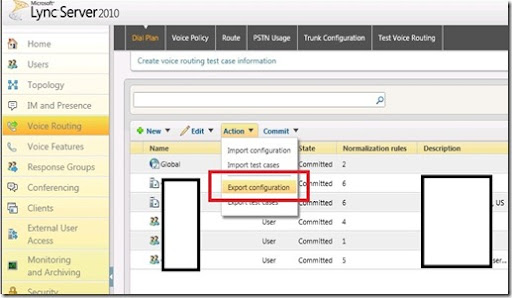When exporting the configuration, it saves as a *.vcfg file.  Using Chris’ handy powershell cmdlet, we can extract the contents of the *.vcfg file into an Excel spreadsheet with different tabs for each area: Dial Plan, Voice Policy, Route, PSTN Usage, and Trunk Configuration.

Copy the following powershell script as a .ps1 file and save in the same location as the exported *.vcfg file.

`##################################################`
`#`
`#  LyncVCFGConverter.ps1`
`#  Format Exported .vcfg file from Lync to Excel`
`#`
`#  Author: Chris Cook`
`#`
`#  Use at your own risk!`
`#`
`##################################################`
` `
` `
`cls`
`#Collect VCFG Filename from Command Line Arguments`
`\$VCFGFileName = \$args`
`Write-Host "Importing XML Config..."`
`\$VCFGXML = [xml](get-content \$VCFGFileName)`
`Write-Host "Done"`
` `
`#Get current working directory and convert it to a string.`
`\$CurrentDirectory = Get-Location`
`\$CurrentDirectory = \$CurrentDirectory.ToString()`
` `
`#Set the file name for the Excel Spreadsheet we're working with.`
`#Drop the VCFG extension and add " - Voice Config.XLSX" to the end.`
`Write-Host "Starting Excel..."`
`\$ExcelFileName = \$VCFGFileName.substring(0,\$VCFGFileName.Length - 5) + " - Voice  Config.XLSX"`
` `
`#Create the Excel Instance we will be working with.`
`\$ExcelApp = New-Object -comobject Excel.Application`
` `
`#Create a new Workbook and add 2 extra sheets for 5 total.`
`Write-Host "Configuring Worksheets..."`
`\$Workbook = \$ExcelApp.Workbooks.Add()`
`\$Worksheets = \$WorkBook.Worksheets.Add()`
`\$Worksheets = \$WorkBook.Worksheets.Add()`
` `
`#Name the sheets in the Workbook and assign variables to them.`
`\$LocationProfilesSheet = \$Workbook.Worksheets.Item(1)`
`\$LocationProfilesSheet.Name = "Location Profiles"`
`\$PSTNUsagesSheet = \$Workbook.Worksheets.Item(2)`
`\$PSTNUsagesSheet.Name = "PSTN Usages"`
`\$PSTNRoutesSheet = \$Workbook.Worksheets.Item(3)`
`\$PSTNRoutesSheet.Name = "PSTN Routes"`
`\$VoicePolicySheet = \$Workbook.Worksheets.Item(4)`
`\$VoicePolicySheet.Name = "Voice Policies"`
`\$TrunkSheet = \$Workbook.Worksheets.Item(5)`
`\$TrunkSheet.Name = "Trunk Configuration"`
`Write-Host "Done"`
` `
`#Configure layout for Location Profile Sheet`
`Write-Host "**Building Location Profile Sheet**"`
`\$LocationProfilesSheet.Cells.Item(1,1) = "Dial Plan"`
`\$LocationProfilesSheet.Cells.Item(1,2) = "Scope"`
`\$LocationProfilesSheet.Cells.Item(1,3) = "Dial Plan Description"`
`\$LocationProfilesSheet.Cells.Item(1,4) = "Rule"`
`\$LocationProfilesSheet.Cells.Item(1,5) = "Rule Description"`
`\$LocationProfilesSheet.Cells.Item(1,6) = "Pattern"`
`\$LocationProfilesSheet.Cells.Item(1,7) = "Translation"`
`\$LocationProfilesSheet.Cells.Item(1,8) = "Dialin Conferencing Region"`
` `
`\$CurrentRow = 2`
` `
`#Import XML data into Excel fields.`
`\$LocationProfiles = @(\$VCFGXML.Datasource.LocationProfiles.Datasource)`
`ForEach (\$DialPlan in \$LocationProfiles){`
`Write-Host "Found Dial Plan: " \$DialPlan.DialPlanName`
`ForEach (\$Rule in \$DialPlan.Rules.Datasource){`
`Write-Host "Importing Rule: " \$Rule.Name`
`\$LocationProfilesSheet.Cells.Item(\$CurrentRow,1) = \$DialPlan.Name`
`\$LocationProfilesSheet.Cells.Item(\$CurrentRow,2) = \$DialPlan.Scope`
`\$LocationProfilesSheet.Cells.Item(\$CurrentRow,3) = \$DialPlan.Description`
`\$LocationProfilesSheet.Cells.Item(\$CurrentRow,4) = \$Rule.Name`
`\$LocationProfilesSheet.Cells.Item(\$CurrentRow,5) = \$Rule.Description`
`\$LocationProfilesSheet.Cells.Item(\$CurrentRow,6) = \$Rule.Pattern`
`\$LocationProfilesSheet.Cells.Item(\$CurrentRow,7) = \$Rule.Translation`
`\$LocationProfilesSheet.Cells.Item(\$CurrentRow,8) = \$DialPlan.DialinConferencingRegion`
`\$CurrentRow = \$CurrentRow + 1`
`}`
`}`
`Write-Host "**Done with Location Profile Sheet**"`
` `
`#Configure layout for PSTN Usages Sheet`
`Write-Host "**Building PSTN Usages Sheet**"`
`\$PSTNUsagesSheet.Cells.Item(1,1) = "Name"`
`\$PSTNUsagesSheet.Cells.Item(1,2) = "Identity"`
`\$PSTNUsagesSheet.Cells.Item(1,3) = "Scope"`
`\$CurrentRow = 2`
` `
`#Import XML data into Excel fields.`
`\$PSTNUsages = @(\$VCFGXML.Datasource.PSTNUsages.Datasource)`
`ForEach (\$Usages in \$PSTNUsages){`
`ForEach (\$Usage in \$Usages.Usages.Datasource){`
`Write-Host "Importing Usage: " \$Usage.Name`
`\$PSTNUsagesSheet.Cells.Item(\$CurrentRow,1) = \$Usage.Name`
`\$PSTNUsagesSheet.Cells.Item(\$CurrentRow,2) = \$Usages.Identity`
`\$PSTNUsagesSheet.Cells.Item(\$CurrentRow,3) = \$Usages.Scope`
`\$CurrentRow = \$CurrentRow + 1`
`}`
`}`
`Write-Host "**Done with PSTN Usages Sheet**"`
` `
`#Configure layout for PSTN Route Settings Sheet`
`Write-Host "**Building PSTN Route Settings Sheet**"`
`\$PSTNRoutesSheet.Cells.Item(1,1) = "Route Name"`
`\$PSTNRoutesSheet.Cells.Item(1,2) = "Usage"`
`\$PSTNRoutesSheet.Cells.Item(1,3) = "Route Description"`
`\$PSTNRoutesSheet.Cells.Item(1,4) = "Number Pattern"`
`\$PSTNRoutesSheet.Cells.Item(1,5) = "Supress Caller ID"`
`\$PSTNRoutesSheet.Cells.Item(1,6) = "PSTN Gateway"`
`\$CurrentRow = 2`
` `
`#Import XML data into Excel fields.`
`\$RouteSettings = @(\$VCFGXML.Datasource.PSTNRouteSettings.Datasource)`
`ForEach (\$RouteGroup in \$RouteSettings){`
`ForEach (\$Route in \$RouteGroup.Routes.Datasource){`
`Write-Host "Importing Route: " \$Route.Name`
`\$PSTNRoutesSheet.Cells.Item(\$CurrentRow,1) = \$Route.Name`
`\$PSTNRoutesSheet.Cells.Item(\$CurrentRow,3) = \$Route.Description`
`\$PSTNRoutesSheet.Cells.Item(\$CurrentRow,4) = \$Route.NumberPattern`
`\$PSTNRoutesSheet.Cells.Item(\$CurrentRow,5) = \$Route.SuppressCallerId`
`ForEach (\$Usage in \$Route.Usages.Datasource){`
`\$PSTNRoutesSheet.Cells.Item(\$CurrentRow,2) = \$Usage.Name`
`}`
`ForEach (\$Gateway in \$Route.Gateways.Datasource){`
`\$PSTNRoutesSheet.Cells.Item(\$CurrentRow,6) = \$Gateway.ServiceId`
`}`
`\$CurrentRow = \$CurrentRow + 1`
`}`
`}`
`Write-Host "**Done with PSTN Route Settings Sheet**"`
` `
`#Configure layout for Voice Policies Sheet`
`Write-Host "**Building Voice Policies Sheet**"`
`\$VoicePolicySheet.Cells.Item(1,1) = "Voice Policy Name"`
`\$VoicePolicySheet.Cells.Item(1,2) = "Description"`
`\$VoicePolicySheet.Cells.Item(1,3) = "Scope"`
`\$VoicePolicySheet.Cells.Item(1,4) = "Usage"`
`\$VoicePolicySheet.Cells.Item(1,5) = "Allow Simultaneous Ring"`
`\$VoicePolicySheet.Cells.Item(1,6) = "Allow Call Forwarding"`
`\$VoicePolicySheet.Cells.Item(1,7) = "Allow PSTN Rerouting"`
`\$VoicePolicySheet.Cells.Item(1,8) = "Enable Delegation"`
`\$VoicePolicySheet.Cells.Item(1,9) = "Enable Team Call"`
`\$VoicePolicySheet.Cells.Item(1,10) = "Enable Call Transfer"`
`\$VoicePolicySheet.Cells.Item(1,11) = "Enable Call Park"`
`\$VoicePolicySheet.Cells.Item(1,12) = "Enable Malicious Call tracing"`
`\$VoicePolicySheet.Cells.Item(1,13) = "Enable Bandwidth Policy Override"`
`\$CurrentRow = 2`
` `
`#Import XML data into Excel fields.`
`\$VoicePolicies = @(\$VCFGXML.Datasource.VoicePolicies.Datasource)`
`ForEach (\$Policy in \$VoicePolicies){`
`Write-Host "Importing Policies for: " \$Policy.Name`
`ForEach (\$Usage in \$Policy.Usages.Datasource){`
`\$VoicePolicySheet.Cells.Item(\$CurrentRow,1) = \$Policy.Name`
`\$VoicePolicySheet.Cells.Item(\$CurrentRow,2) = \$Policy.Description`
`\$VoicePolicySheet.Cells.Item(\$CurrentRow,3) = \$Policy.Scope`
`\$VoicePolicySheet.Cells.Item(\$CurrentRow,4) = \$Usage.Name`
`\$VoicePolicySheet.Cells.Item(\$CurrentRow,5) = \$Policy.AllowSimulRing`
`\$VoicePolicySheet.Cells.Item(\$CurrentRow,6) = \$Policy.AllowCallForwarding`
`\$VoicePolicySheet.Cells.Item(\$CurrentRow,7) = \$Policy.AllowPSTNReRouting`
`\$VoicePolicySheet.Cells.Item(\$CurrentRow,8) = \$Policy.EnableDelegation`
`\$VoicePolicySheet.Cells.Item(\$CurrentRow,9) = \$Policy.EnableTeamCall`
`\$VoicePolicySheet.Cells.Item(\$CurrentRow,10) = \$Policy.EnableCallTransfer`
`\$VoicePolicySheet.Cells.Item(\$CurrentRow,11) = \$Policy.EnableCallPark`
`\$VoicePolicySheet.Cells.Item(\$CurrentRow,12) = \$Policy.EnableMaliciousCallTracing`
`\$VoicePolicySheet.Cells.Item(\$CurrentRow,13) = \$Policy.EnableBWPolicyOverride`
`\$CurrentRow = \$CurrentRow + 1`
`}`
`}`
`Write-Host "**Done with Voice Policies Sheet**"`
` `
`#Configure layout for Trunks Sheet`
`Write-Host "**Building Trunk Sheet**"`
`\$TrunkSheet.Cells.Item(1,1) = "Trunk Name"`
`\$TrunkSheet.Cells.Item(1,2) = "Trunk Identity"`
`\$TrunkSheet.Cells.Item(1,3) = "Scope"`
`\$TrunkSheet.Cells.Item(1,4) = "Rule"`
`\$TrunkSheet.Cells.Item(1,5) = "Rule Description"`
`\$TrunkSheet.Cells.Item(1,6) = "Concentrated Topology"`
`\$TrunkSheet.Cells.Item(1,7) = "Enable Bypass"`
`\$TrunkSheet.Cells.Item(1,8) = "Enable Refer Support"`
`\$TrunkSheet.Cells.Item(1,9) = "SRTP Mode"`
`\$TrunkSheet.Cells.Item(1,10) = "Max. Early Dialogs"`
`\$TrunkSheet.Cells.Item(1,11) = "Pattern"`
`\$TrunkSheet.Cells.Item(1,12) = "Translation"`
`\$CurrentRow = 2`
` `
`#Import XML data into Excel fields.`
`\$TrunkGroup = @(\$VCFGXML.Datasource.Trunks.Datasource)`
`ForEach (\$Trunk in \$TrunkGroup){`
`Write-Host "Found Trunk: " \$Trunk.Name`
`ForEach (\$Rule in \$Trunk.Rules.Datasource){`
`Write-Host "Importing Rule: " \$Rule.Name`
`\$TrunkSheet.Cells.Item(\$CurrentRow,1) = \$Trunk.Name`
`\$TrunkSheet.Cells.Item(\$CurrentRow,2) = \$Trunk.Identity`
`\$TrunkSheet.Cells.Item(\$CurrentRow,3) = \$Trunk.Scope`
`\$TrunkSheet.Cells.Item(\$CurrentRow,4) = \$Rule.Name`
`\$TrunkSheet.Cells.Item(\$CurrentRow,5) = \$Rule.Description`
`\$TrunkSheet.Cells.Item(\$CurrentRow,6) = \$Trunk.ConcentratedTopology`
`\$TrunkSheet.Cells.Item(\$CurrentRow,7) = \$Trunk.EnableBypass`
`\$TrunkSheet.Cells.Item(\$CurrentRow,8) = \$Trunk.EnableReferSupport`
`\$TrunkSheet.Cells.Item(\$CurrentRow,9) = \$Trunk.SRTPMode`
`\$TrunkSheet.Cells.Item(\$CurrentRow,10) = \$Trunk.MaxEarlyDialogs`
`\$TrunkSheet.Cells.Item(\$CurrentRow,11) = \$Rule.Pattern`
`\$TrunkSheet.Cells.Item(\$CurrentRow,12) = \$Rule.Translation`
`\$CurrentRow = \$CurrentRow + 1`
`}`
`}`
`Write-Host "**Done with Voice Policies Sheet**"`
` `
` `
` `
` `
` `
`#Make Excel visible to the user.`
`\$ExcelApp.Visible = \$True`
`\$Workbook.SaveAs(\$CurrentDirectory + "\" + \$ExcelFileName)`
` `
`#\$ExcelApp.Quit()`

Now open up a powershell command window and navigate to the directory with the .ps1 file and .vcfg file and run the following command:

.\LyncVCFGConverter.ps1 CustomerA_Lync.vcfg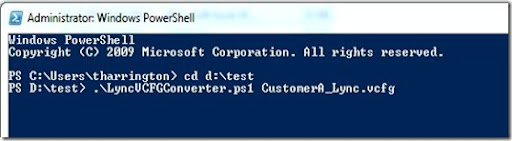The script will extract the data and create an Excel spreadsheet.  The progress is shown as the script is running: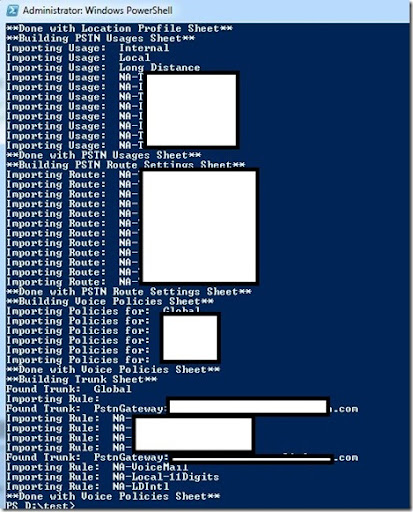Once the script is complete, the Excel spreadsheet will be saved and launched.  You will notice that the spreadsheet is divided into different tabs: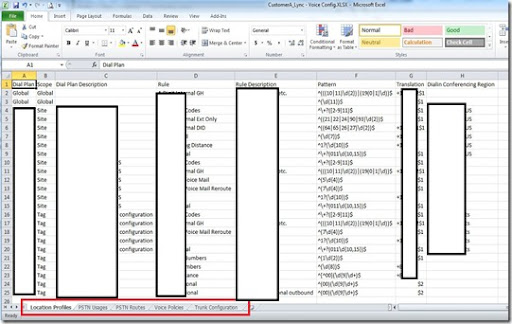Here are some examples from some of the other tabs…

Voice Policies: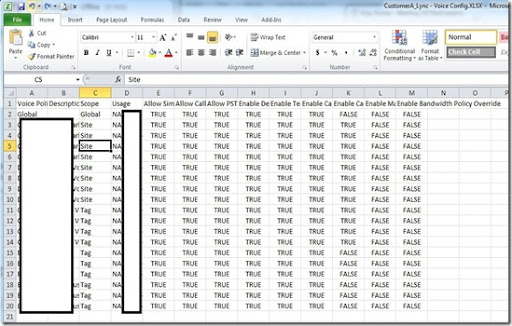Trunk Configuration: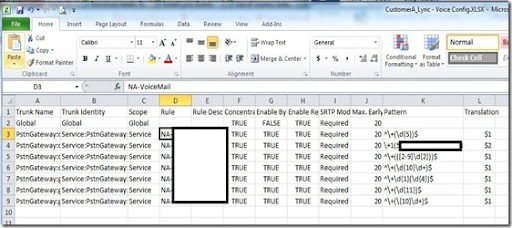Hopefully this will help you out on documenting your Lync Voice configurations!  Again, thanks to Chris Cook for the heavy lifting on the powershell scripts.